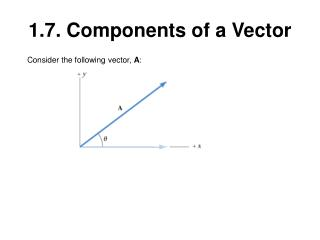DownloadDownload Presentation1.7. Components of a Vector

# 1.7. Components of a Vector

Download Presentation## 1.7. Components of a Vector

- - - - - - - - - - - - - - - - - - - - - - - - - - - E N D - - - - - - - - - - - - - - - - - - - - - - - - - - -
##### Presentation Transcript

1. 1.7. Components of a Vector Consider the following vector, A:

2. 1.7. Components of a Vector Consider the following vector, A: How can we replace vector A by two perpendicular components?

3. 1.7. Components of a Vector

4. 1.7. Components of a Vector Aadj=A∙Cos θ and Aopp=A∙Sin θ

5. Problem-39 The drawing shows a force vector that has a magnitude of 475 N. Find the (a) x, (b) y, and (c) z components of the vector.

6. Vector Components A jogger runs 145 m in a direction 20.0° east of north (displacement vector A) and then 105 m in a direction 35.0° south of east (displacement vector B).

7. Problem Find the resultant of the three displacement vectors in the drawing by means of the component method. The magnitudes of the vectors are A = 5.00 m, B = 5.00 m, and C = 4.00 m.